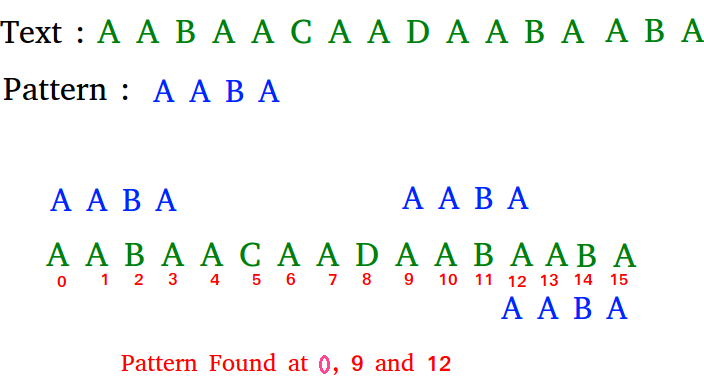Java Program for Rabin-Karp Algorithm for Pattern Searching

• Last Updated : 11 Dec, 2018
Given a text txt[0..n-1] and a pattern pat[0..m-1], write a function search(char pat[], char txt[]) that prints all occurrences of pat[] in txt[]. You may assume that n > m.

Examples:

Input:  txt[] = "THIS IS A TEST TEXT"
pat[] = "TEST"
Output: Pattern found at index 10

pat[] =  "AABA"
Output: Pattern found at index 0
Pattern found at index 9
Pattern found at index 12The Naive String Matching algorithm slides the pattern one by one. After each slide, it one by one checks characters at the current shift and if all characters match then prints the match.
Like the Naive Algorithm, Rabin-Karp algorithm also slides the pattern one by one. But unlike the Naive algorithm, Rabin Karp algorithm matches the hash value of the pattern with the hash value of current substring of text, and if the hash values match then only it starts matching individual characters. So Rabin Karp algorithm needs to calculate hash values for following strings.

1) Pattern itself.
2) All the substrings of text of length m.

Java

 // Following program is a Java implementation// of Rabin Karp Algorithm given in the CLRS book  public class Main {    // d is the number of characters in the input alphabet    public final static int d = 256;      /* pat -> pattern        txt -> text        q -> A prime number    */    static void search(String pat, String txt, int q)    {        int M = pat.length();        int N = txt.length();        int i, j;        int p = 0; // hash value for pattern        int t = 0; // hash value for txt        int h = 1;          // The value of h would be "pow(d, M-1)%q"        for (i = 0; i < M - 1; i++)            h = (h * d) % q;          // Calculate the hash value of pattern and first        // window of text        for (i = 0; i < M; i++) {            p = (d * p + pat.charAt(i)) % q;            t = (d * t + txt.charAt(i)) % q;        }          // Slide the pattern over text one by one        for (i = 0; i <= N - M; i++) {              // Check the hash values of current window of text            // and pattern. If the hash values match then only            // check for characters on by one            if (p == t) {                /* Check for characters one by one */                for (j = 0; j < M; j++) {                    if (txt.charAt(i + j) != pat.charAt(j))                        break;                }                  // if p == t and pat[0...M-1] = txt[i, i+1, ...i+M-1]                if (j == M)                    System.out.println("Pattern found at index " + i);            }              // Calculate hash value for next window of text: Remove            // leading digit, add trailing digit            if (i < N - M) {                t = (d * (t - txt.charAt(i) * h) + txt.charAt(i + M)) % q;                  // We might get negative value of t, converting it                // to positive                if (t < 0)                    t = (t + q);            }        }    }      /* Driver program to test above function */    public static void main(String[] args)    {        String txt = "GEEKS FOR GEEKS";        String pat = "GEEK";        int q = 101; // A prime number        search(pat, txt, q);    }}  // This code is contributed by nuclode
Output:
Pattern found at index 0
Pattern found at index 10

Please refer complete article on Rabin-Karp Algorithm for Pattern Searching for more details!

My Personal Notes arrow_drop_up